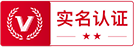## 土地估价师## 税务师# 2020年01月02日中药学综合知识与技能试题(第 1 套 - A型)

※中药学综合知识与技能在线模考>>开始

■ A型题

1. 下列表述中属于症的是
• A.消渴
• B.恶寒
• C.肺痈
• D.水肿
• E.咳嗽

2. 下述疾病现象中属于阴的是
• A.面色鲜明
• B.咳声有力
• C.脉象滑数
• D.声低气微
• E.脉象洪大

3. 临床常见的心火引动肝火的病证，属于
• A.相乘传变
• B.子病犯母
• C.母病及子
• D.反克传变
• E.相侮传变

4. “肝肾同源”的主要理论依据是
• A.同居下焦
• B.藏泄互用
• C.精血互化
• D.阴液互补
• E.阴阳承制

5. “血之余”是指
• A.髓
• B.齿
• C.爪
• D.发
• E.筋

6. 肺进行一切生理活动的基础是
• A.肺气的宣发肃降运动
• B.肺主呼吸之气
• C.肺主营气的生成
• D.肺主调节全身气机
• E.肺主治节

7. 成人牙齿松动、过早脱落的根本原因在于
• A.肾阳亏乏
• B.命门虚寒
• C.肾精亏损
• D.肾阴虚衰
• E.肾气不固

8. 心对血液的主要作用是
• A.藏血
• B.统血
• C.行血
• D.防止出血
• E.调节血量

9. 体内液态物质的运行、输布和排泄，主要依赖气的哪些功能配合
• A.推动与温煦
• B.防御与固摄
• C.推动与固摄
• D.中介与推动
• E.温煦与凉润

10. 推动人体生长发育及脏腑功能活动的气是
• A.元气
• B.宗气
• C.营气
• D.卫气
• E.肝气

11. 头痛的部位在前额者，病变多在
• A.厥阴经
• B.阳明经
• C.太阳经
• D.少阳经
• E.少阴经

12. 经络系统中，与脏腑有直接络属关系的是
• A.十二经筋
• B.十二经别
• C.十二皮部
• D.奇经八脉
• E.十二经脉

13. 素体阳虚畏寒者，易致邪从
• A.寒化
• B.热化
• C.燥化
• D.湿化
• E.火化

14. 痰致病广泛，变化多端的原因是
• A.痰可扰乱神明
• B.痰可化火化风
• C.痰阻碍气血运行
• D.痰似风善行数变
• E.痰可随气升降无处不到

15. 在六淫中，最易伤肺的邪气是
• A.风邪
• B.寒邪
• C.暑邪
• D.湿邪
• E.燥邪

16. 在一定条件下，“五志”和“五气”皆可化
• A.风
• B.寒
• C.湿
• D.火
• E.燥

17. 七情太过首先伤及
• A.肝气
• B.脾阳
• C.肾精
• D.肺津
• E.心神

18. 以下不属于阴偏盛的病理变化的是
• A.真寒假热
• B.阴盛格阳
• C.阴胜则寒
• D.虚寒证
• E.阴胜则阳病

19. 从虚实角度看，“阴胜则阳病”属于
• A.真虚假实
• B.真实假虚
• C.由实转虚
• D.由虚转实
• E.虚实错杂

20. 脾气虚损，运化无力，导致水湿内停的病理变化属于
• A.真虚假实
• B.实中夹虚
• C.虚中夹实
• D.因虚致实
• E.由实转虚

21. 患者先有阴虚内热病证，后又出现畏寒肢冷，大便溏泄，其病机应是
• A.阴损及阳
• B.阳损及阴
• C.阴阳亡失
• D.阳盛格阴
• E.阴盛格阳

22. “治未病”是指
• A.防止疾病的发生和发展
• B.外避病邪和既病防变
• C.未病先防和早期诊治
• D.未病先防和既病防变
• E.调养正气和控制疾病传变

23. 以下脉象不属轻取即得的是
• A.浮脉
• B.濡脉
• C.散脉
• D.牢脉
• E.芤脉

24. 温病患者热入营血时，舌色应为
• A.红舌
• B.绛舌
• C.淡白舌
• D.青舌
• E.淡红舌

25. 半表半里证的寒热特点是
• A.恶寒重发热轻
• B.发热重恶寒轻
• C.发热轻而恶风
• D.寒热往来
• E.寒热俱重

26. 下列诸脉中，一般不主痰饮证的脉象是
• A.滑脉
• B.弦脉
• C.促脉
• D.结脉
• E.濡脉

27. 喘证的临床表现应除外哪一项
• A.呼吸困难
• B.鼻翼扇动
• C.张口抬肩
• D.难以平卧
• E.喉中痰鸣

28. 寒凝血瘀患者唇色
• A.淡白
• B.樱桃红
• C.深红
• D.青紫
• E.青黑

29. 下列哪项可出现“胃不和则卧不安”
• A.心肾不交
• B.痰湿困脾
• C.胆郁痰扰
• D.食滞内停
• E.心脾两虚

30. 痛如针刺的病机是
• A.食积
• B.气滞
• C.湿滞
• D.瘀血
• E.血虚

31. 心气虚的临床表现是
• A.心悸气短，神疲乏力
• B.心悸气短，眩晕健忘
• C.心悸气短，胸闷汗出
• D.心悸气短，胸痛彻背
• E.心悸气短，痰涎壅盛

32. 咳嗽，咯黄稠痰，鼻流浊涕，口干咽痛，身热，微恶风寒者，应诊为
• A.风热犯肺证
• B.燥邪犯肺证
• C.肺肾阴虚证
• D.痰浊阻肺证
• E.肺脾两虚证

33. 肝胆湿热证的临床表现不见
• A.舌红苔黄腻
• B.脉弦细数
• C.外阴瘙痒
• D.右胁胀痛
• E.黄疸

34. 阳水一般先见
• A.头顶水肿
• B.颈项水肿
• C.满面水肿
• D.下肢水肿
• E.眼睑水肿

35. 血热证的临床表现常见
• A.局部肿胀刺痛
• B.各种出血证
• C.颧红
• D.心悸
• E.口干但欲漱水不欲咽

36. 眩晕欲仆，头胀头痛，急躁易怒，步履不稳，属
• A.肝阳上亢证
• B.肝风内动证
• C.热极生风证
• D.血虚生风证
• E.肝血虚证

37. 患者渴喜冷饮、烦躁不安、便干尿黄、舌红苔黄，同时又见四肢厥冷、脉沉等症，应采用的治法是
• A.缓则治本
• B.急则治标
• C.虚则补之
• D.寒者热之
• E.寒因寒用

38. 眩晕兼见面红目赤，耳鸣，口苦尿黄，胁肋胀痛，脉弦数，证属
• A.气血亏虚
• B.肝火上炎
• C.痰浊上蒙
• D.瘀血阻窍
• E.肝肾阴虚

39. 气滞血瘀型痛经首选
• A.少腹逐瘀汤
• B.膈下逐瘀汤
• C.血府逐瘀汤
• D.金匮温经汤
• E.胶艾四物汤

40. 产生苦味的五元是
• A.土与水
• B.火与土
• C.水与火
• D.水与风
• E.火与风

41. 赫依属五元之
• A.火
• B.土
• C.气
• D.水
• E.空

42. 称为众方之祖的医学典籍是
• A.《伤寒论》
• B.《温疫论》
• C.《千金翼方》
• D.《黄帝内经》
• E.《肘后备急方》

43. 中国古代收方最多的方书是
• A.《普济方》
• B.《千金要方》
• C.《千金翼方》
• D.《和剂局方》
• E.《太平圣惠方》

44. 下面哪一项内容是医师咨询最多的问题
• A.不良反应
• B.新药信息
• C.合理用药信息
• D.禁忌证
• E.药品价格

45. 医生对饮片修治提出要求药名是
• A.远志去心
• B.酒蒸大黄
• C.蜜炙麻黄
• D.东阿胶
• E.马蹄决明

46. 抗疟药宜
• A.空腹服
• B.饭前服
• C.饭后服
• D.发作前1～2小时服
• E.睡觉前服

47. 大腹子的正名是
• A.香加皮
• B.青皮
• C.泽泻
• D.槟榔
• E.桑白皮

48. 功效不同，不可同放于一个斗中的是
• A.板蓝根和大青叶
• B.当归和川芎
• C.萹蓄和瞿麦
• D.当归和独活
• E.牡丹皮和赤芍

49. 煎后药渣挤出的残液应不超出药渣总量的
• A.5％
• B.10％
• C.15％
• D.20％
• E.25％

50. 罂粟壳每张处方用量规定为
• A.3～6g
• B.5～8g
• C.8～15g
• D.15～18g
• E.18～20g

51. 最易霉变的剂型是
• A.丸剂
• B.合剂
• C.酊剂
• D.针剂
• E.糖浆剂

52. 药品不宜长时间贮存应做到
• A.易变后出
• B.细贵后出
• C.易燃后出
• D.先产先出
• E.近效期后出

53. 下列易泛油的中药饮片是
• A.大黄
• B.黄柏
• C.黄芪
• D.白芍
• E.当归

54. 动物类药物如蛇类、虫类等宜与共贮的有
• A.花椒
• B.大枣
• C.山茱萸
• D.五味子
• E.决明子

55. 下列各组联用不合理的是
• A.金匮肾气丸与牛黄解毒片
• B.益母草颗粒与妇血康颗粒
• C.附子理中丸与健脾丸
• D.牛黄清心丸与柏子养心丸
• E.金锁固精丸与参苓白术丸

56. 中西药联用可以降低西药用药剂量的药组是
• A.石麦汤与氯氮平
• B.丹参注射液与多巴胺
• C.苓桂术甘汤与地西泮
• D.蛤蚧定喘丸与异烟肼
• E.女金丹与诺氟沙星

57. 不能与海藻、昆布合用的是
• A.硝酸甘油
• B.镇咳药
• C.治疗甲状腺功能亢进的西药
• D.三环类抗抑郁药
• E.降糖西药

58. 地西泮与苓桂术甘汤联用，地西泮用量只需常规用量的
• A.1／2
• B.1／3
• C.1／4
• D.1／5
• E.1／6

59. 消渴丸中含的西药成分是
• A.格列苯脲
• B.格列本脲
• C.吲哚美辛
• D.苯甲酸
• E.水杨酸

60. 含马钱子的中成药是
• A.追风丸
• B.舒筋丸
• C.紫金锭
• D.祛风止痛片
• E.三七伤药片

※中药学综合知识与技能在线模考>>查看答案
 留言与评论（共有 条评论）

 验证码：http://www.zuoti.com/

### var _hmt = _hmt || []; (function() { var hm = document.createElement("script"); hm.src = "https://hm.baidu.com/hm.js?db7dc45ce37deaf748e02c0394b4349b"; var s = document.getElementsByTagName("script"); s.parentNode.insertBefore(hm, s); })(); | 闽ICP备20005157号-1